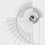# The Special Number 3816547290

3816547290

A Special Number: the only one in which

Every digit is used,

Each digit is used only once, and

The first n digits are divisible by n, for n=1..10.


3 is divisible by 1,

38 is divisible by 2,

381 is divisible by 3,

3816 is divisible by 4,

38165 is divisible by 5,

381654 is divisible by 6,

3816547 is divisible by 7,

38165472 is divisible by 8,

381654729 is divisible by 9,

3816547290 is divisible by 10.Note by Faizan Khan
5 years, 10 months ago

This discussion board is a place to discuss our Daily Challenges and the math and science related to those challenges. Explanations are more than just a solution — they should explain the steps and thinking strategies that you used to obtain the solution. Comments should further the discussion of math and science.

When posting on Brilliant:

• Use the emojis to react to an explanation, whether you're congratulating a job well done , or just really confused .
• Ask specific questions about the challenge or the steps in somebody's explanation. Well-posed questions can add a lot to the discussion, but posting "I don't understand!" doesn't help anyone.
• Try to contribute something new to the discussion, whether it is an extension, generalization or other idea related to the challenge.
• Stay on topic — we're all here to learn more about math and science, not to hear about your favorite get-rich-quick scheme or current world events.

MarkdownAppears as
*italics* or _italics_ italics
**bold** or __bold__ bold
- bulleted- list
• bulleted
• list
1. numbered2. list
1. numbered
2. list
Note: you must add a full line of space before and after lists for them to show up correctly
paragraph 1paragraph 2

paragraph 1

paragraph 2

[example link](https://brilliant.org)example link
> This is a quote
This is a quote
    # I indented these lines
# 4 spaces, and now they show
# up as a code block.

print "hello world"
# I indented these lines
# 4 spaces, and now they show
# up as a code block.

print "hello world"
MathAppears as
Remember to wrap math in $$ ... $$ or $ ... $ to ensure proper formatting.
2 \times 3 $2 \times 3$
2^{34} $2^{34}$
a_{i-1} $a_{i-1}$
\frac{2}{3} $\frac{2}{3}$
\sqrt{2} $\sqrt{2}$
\sum_{i=1}^3 $\sum_{i=1}^3$
\sin \theta $\sin \theta$
\boxed{123} $\boxed{123}$

## Comments

Sort by:

Top Newest

Good job, Falzan!

- 5 years, 9 months ago

Log in to reply

i've always been intersred in relation between numbers .... awesome !!

- 5 years, 10 months ago

Log in to reply

By the way I already knew that number.

- 5 years, 10 months ago

Log in to reply

Wow, beautiful!

- 5 years, 10 months ago

Log in to reply

That's brilliant

- 5 years, 10 months ago

Log in to reply

On Brilliant there are question related to this number. This number can found out without hit-n-trial method.

Clearly the 5th and 10th digits are 5 & 0 respectively,

Now 4th digit can only be from 2 & 6 as 3rd digit is odd and for the number to be divisible by 4 its last two digits must be divisible by 4,

Sum of first 3, next 3 & next 3 digits must be divisible by 3,

Hence,

We get the following combinations for 4th,5th &6th digits - 258 or 654,

Now with use of divisibility of 8 we can figure out 6th,7th & 8th digits,

With help of less hard work we can figure out the number.

- 5 years, 10 months ago

Log in to reply

I would like to clarify your solution-We know that a number is divisible by $4$ when last two digits are divisible by $4$ and there are no two digit numbers having $4$ and $8$ as last digits and are divisible by $4$ therefore for $4^{th}$ digit we can say that at the $4^{th}$ place only $2$ or $4$ are possible digits.

Similarly , as $8$ is divisible by $4$ the $8^{th}$ digit would be either $2$ or $4$. Then, as $Akshay$ told we can proceed to get the answer.

- 5 years, 10 months ago

Log in to reply

Hmmm... Well answered brother.. Thankss

- 5 years, 10 months ago

Log in to reply

Great Work.. Its Awesome// how did you found it?

- 5 years, 10 months ago

Log in to reply

×

Problem Loading...

Note Loading...

Set Loading...